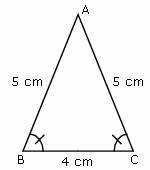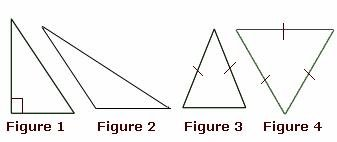Definition Of Isosceles Triangle

When the two sides of a triangle are equal, then the triangle is called as an Isosceles Triangle.

The diagonals of an Isosceles Triangle are equal.
In an isosceles triangle, the two angles are equal.

Example of Isosceles Triangleâ–³ABC is an isosceles triangle as sides AB and AC are equal and also ∠ABC and ∠BCA are equal.

Solved Example on Isosceles Triangle

Ques: Which of the following is an isosceles triangle?A. Figure 2
B. Figure 4
C. Figure 1
D. Figure 3